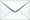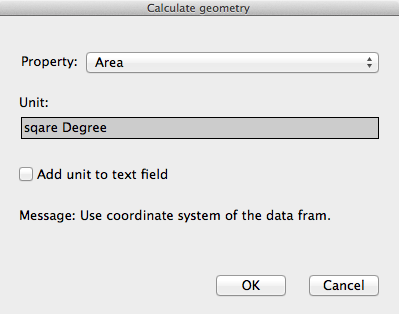Product Search

Dear customers:

take action as soon as possible.tyevolution@yahoo.com

# EC: Attribute table__Calculate geometry

Views:74times

The Calculate Geometry tool allows you to access the geometry of the features in a layer. The tool can calculate coordinate values, lengths, and areas, depending on the geometry of the input layer.

The geometric properties you can calculate for different types of features:

• Point features: X coordinate of point, Y coordinate of point

• Line feature: Length 2D, Length 3D, X coordinate of line start, Y coordinate of line start, X coordinate of line end, Y coordinate of line end

• Polygon feature: Area, Perimeter 2D, Perimeter 3D, X coordinate of centroid 2D, Y coordinate of centroid 2D

Steps:

1. Right-click the field heading for which you want to make a calculation and click Calculate Geometry.

2. Click the geometric property you want to calculate.

Different properties are available depending on the type of layer you're using.

3. Click the units of the output calculations.

4. Click [OK].